Include your answers in this document in the sections below the rubric.

# Rubric

Answer the questions with the two data examples.

# Mechanics of a hypothesis test (review)

1. Set up the null and alternative hypotheses in words and notation.

• In words: The population mean for [what is being studied] is different from [value of $$\mu_0$$].’’ (Note that the statement in words is in terms of the alternative hypothesis.)
• In notation: $$H_0: \mu=\mu_0$$ versus $$H_A: \mu \ne \mu_0$$ (where $$\mu_0$$ is specified by the context of the problem).
2. Choose the significance level of the test, such as $$\alpha=0.05$$.

3. Compute the test statistic, such as $$t_{s} = \frac{\bar{Y}-\mu_0}{SE_{\bar{Y}}}$$, where $$SE_{\bar{Y}}=s/\sqrt{n}$$ is the standard error.

4. Determine the tail(s) of the sampling distribution where the $$p$$-value from the test statistic will be calculated (for example, both tails, right tail, or left tail). (Historically, we would compare the observed test statistic, $$t_{s}$$, with the critical value $$t_{\textrm{crit}}=t_{\alpha/2}$$ in the direction of the alternative hypothesis from the $$t$$-distribution table with degrees of freedom $$df = n-1$$.)

5. State the conclusion in terms of the problem.

• Reject $$H_0$$ in favor of $$H_A$$ if $$p\textrm{-value} < \alpha$$.
• Fail to reject $$H_0$$ if $$p\textrm{-value} \ge \alpha$$. (Note: We DO NOT accept $$H_0$$.)
6. Check assumptions of the test (next week).

# Height data for our class

Is the population mean height of UNM students eligible to take Stat 427/527 different from the US average for men (5 ft 9 1/2 in) or women (5 ft 4 in)?

library(tidyverse)
## -- Attaching packages --------------------------------------------------------------------------------------------------------------------------------------------------------- tidyverse 1.2.1 --
## v ggplot2 3.2.1     v purrr   0.3.2
## v tibble  2.1.3     v dplyr   0.8.3
## v tidyr   1.0.0     v stringr 1.4.0
## v readr   1.3.1     v forcats 0.4.0
## -- Conflicts ------------------------------------------------------------------------------------------------------------------------------------------------------------ tidyverse_conflicts() --
## x dplyr::lag()    masks stats::lag()
# install.packages("gsheet")

# Height vs Hand Span
library(gsheet)
dat.hand <- gsheet2tbl(dat.hand.url)
dat.hand <- as.data.frame(dat.hand)
dat.hand <- na.omit(dat.hand)
dat.hand$Gender_M_F <- factor(dat.hand$Gender_M_F, levels = c("F", "M"))

str(dat.hand)
## 'data.frame':    82 obs. of  5 variables:
##  $Table : num 1 1 1 2 2 2 2 2 2 3 ... ##$ Person     : num  1 2 3 1 2 3 4 5 6 1 ...
##  $Gender_M_F : Factor w/ 2 levels "F","M": 2 1 2 1 1 1 2 2 2 1 ... ##$ Height_in  : num  66 65 74.5 64.5 60 63 74 69 67 63 ...
##  $HandSpan_cm: num 21 19 23.5 19.5 17.5 17.5 23.5 21 23 19.5 ... ## - attr(*, "spec")= ## .. cols( ## .. Table = col_double(), ## .. Person = col_double(), ## .. Gender_M_F = col_character(), ## .. Height_in = col_double(), ## .. HandSpan_cm = col_double() ## .. ) ## - attr(*, "na.action")= 'omit' Named int 4 5 6 7 8 9 16 17 18 23 ... ## ..- attr(*, "names")= chr "4" "5" "6" "7" ... Plot the estimated mean from our class sample versus the true US mean. ## If we create a summary data.frame with a similar structure as our data, then we ## can annotate our plot with those summaries. # calculate the estimated mean and order M then F est.mean <- as.numeric(by(dat.hand$Height_in, dat.hand$Gender_M_F, mean)) # combine true US mean with our estimated mean height.true <- data.frame(Gender_M_F = rev(unique(dat.hand$Gender_M_F))
, Height_in = c(64, 69.5, est.mean)
, TrueEst = c(rep("True", 2), rep("Est", 2)))
height.true
##   Gender_M_F Height_in TrueEst
## 1          F  64.00000    True
## 2          M  69.50000    True
## 3          F  65.36974     Est
## 4          M  70.72159     Est

Here’s two ways to plot our data, annotating the observed and hypothesized means.

#$library(ggplot2) p <- ggplot(data = dat.hand, aes(x = Gender_M_F, y = Height_in)) p <- p + geom_boxplot(alpha = 1/4) p <- p + geom_jitter(position = position_jitter(width = 0.1)) p <- p + geom_point(data = height.true, aes(colour = TrueEst, shape = TrueEst), size = 4, alpha = 3/4) print(p)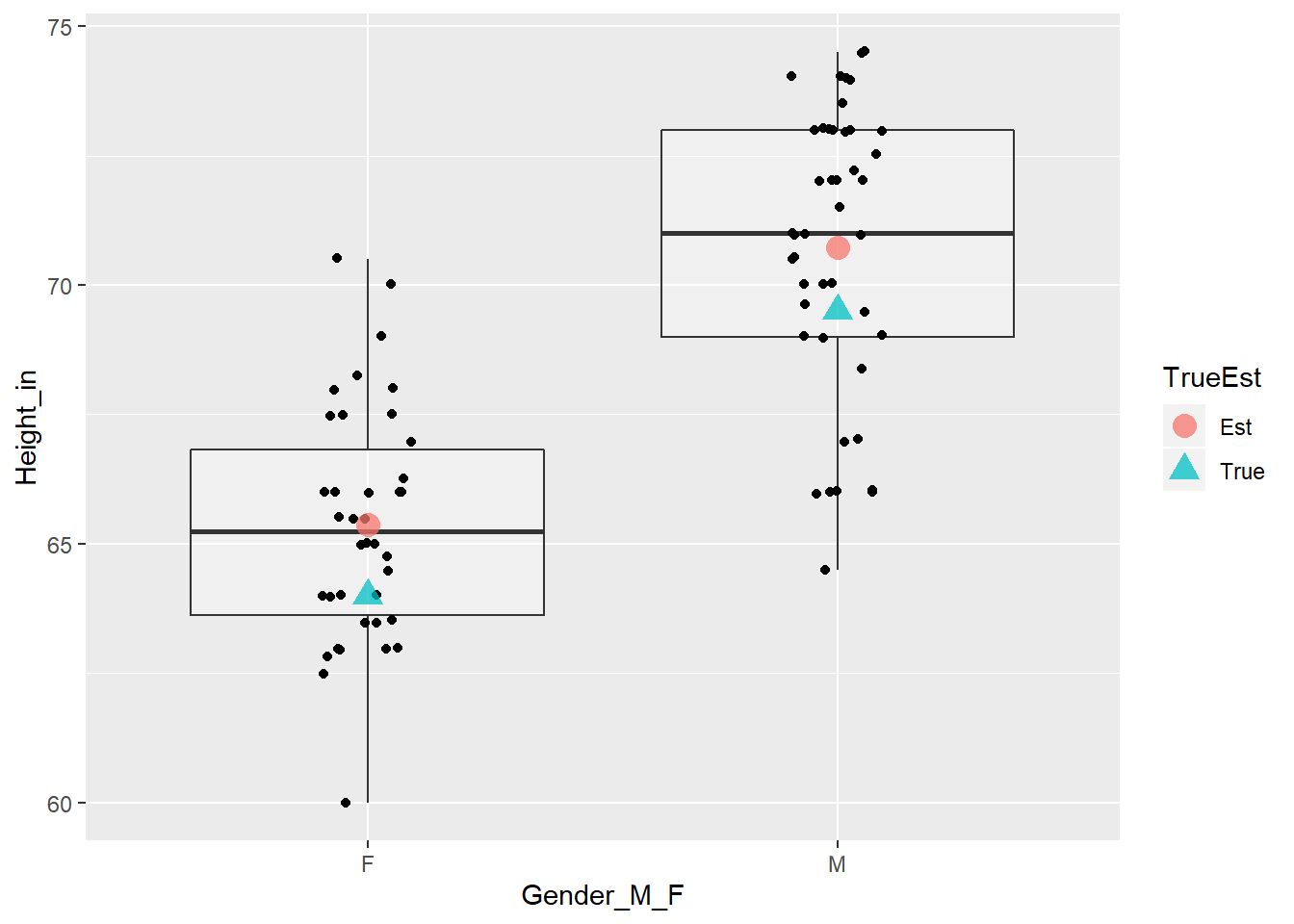library(ggplot2) p <- ggplot(data = dat.hand, aes(x = Height_in)) p <- p + geom_histogram(binwidth = 1) p <- p + geom_vline(data = height.true, aes(xintercept = Height_in, colour = TrueEst, linetype = TrueEst)) p <- p + facet_grid(Gender_M_F ~ .) print(p)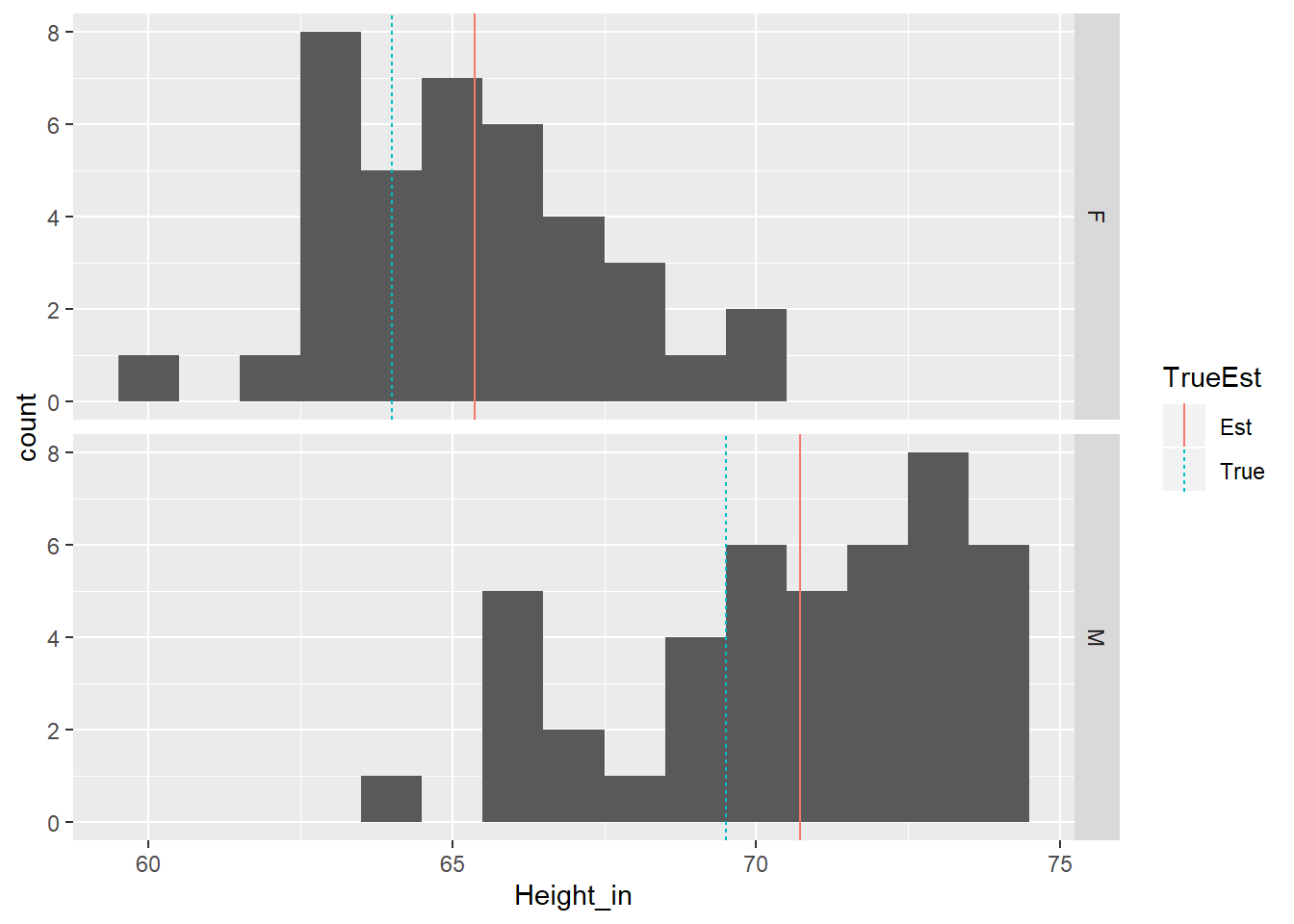## Conduct the hypothesis tests # look at help for t.test # ?t.test # defaults include: alternative = "two.sided", conf.level = 0.95 (I’ve hidden some code from Chapter 02 defining a function to plot the t-distribution with shaded p-value.) ### Test female height equal to US (model example). # test females t.summary.F <- t.test(subset(dat.hand, Gender_M_F == "F", Height_in) , mu = 64) t.summary.F ## ## One Sample t-test ## ## data: subset(dat.hand, Gender_M_F == "F", Height_in) ## t = 3.7475, df = 37, p-value = 0.0006084 ## alternative hypothesis: true mean is not equal to 64 ## 95 percent confidence interval: ## 64.62915 66.11032 ## sample estimates: ## mean of x ## 65.36974 names(t.summary.F) ##  "statistic" "parameter" "p.value" "conf.int" "estimate" ##  "null.value" "stderr" "alternative" "method" "data.name" t.dist.pval(t.summary.F)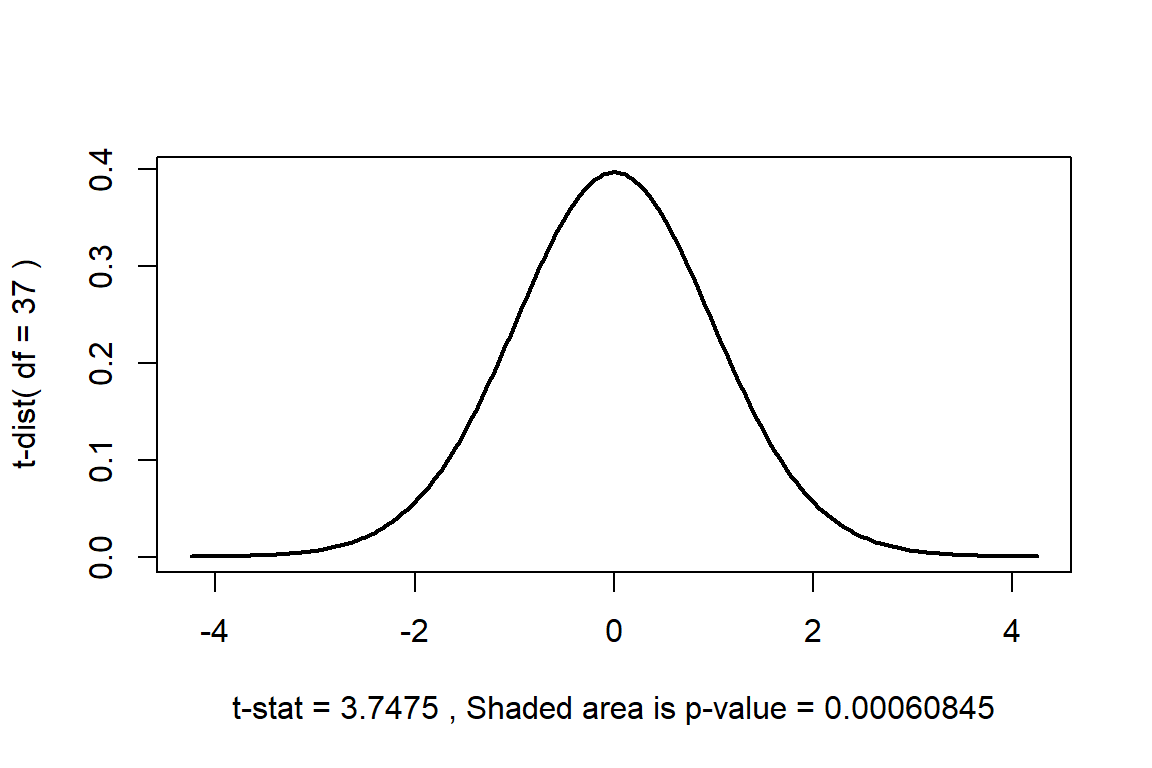Hypothesis test 1. The population mean height for females at UNM eligible to take Stat 427/527 is different from the US population value of $$\mu_0=64$$ inches.’’ • $$H_0: \mu=64$$ versus $$H_A: \mu \ne 64$$ 2. Let $$\alpha=0.05$$, the significance level of the test and the Type-I error probability if the null hypothesis is true. 3. $$t_{s} = 3.748$$. 4. $$p=6.08\times 10^{-4}$$, this is the observed significance of the test. 5. Because $$p=6.08\times 10^{-4} < 0.05$$, we have sufficient evidence to reject $$H_0$$, concluding that the observed mean height is different than the US population mean. ## Questions to answer 1. (3 p) As above, set up the hypothesis test for males, but whether UNM males are taller on average than the US population. ### Test male height greater than US (your turn). ## You'll need to modify the statement below to correspond ## to the hypothesis you wish to test # test males t.summary.M <- t.test(subset(dat.hand, Gender_M_F == "M", Height_in) , mu = 0 , alternative = "two.sided") t.summary.M ## ## One Sample t-test ## ## data: subset(dat.hand, Gender_M_F == "M", Height_in) ## t = 170.6, df = 43, p-value < 2.2e-16 ## alternative hypothesis: true mean is not equal to 0 ## 95 percent confidence interval: ## 69.88560 71.55758 ## sample estimates: ## mean of x ## 70.72159 t.dist.pval(t.summary.M)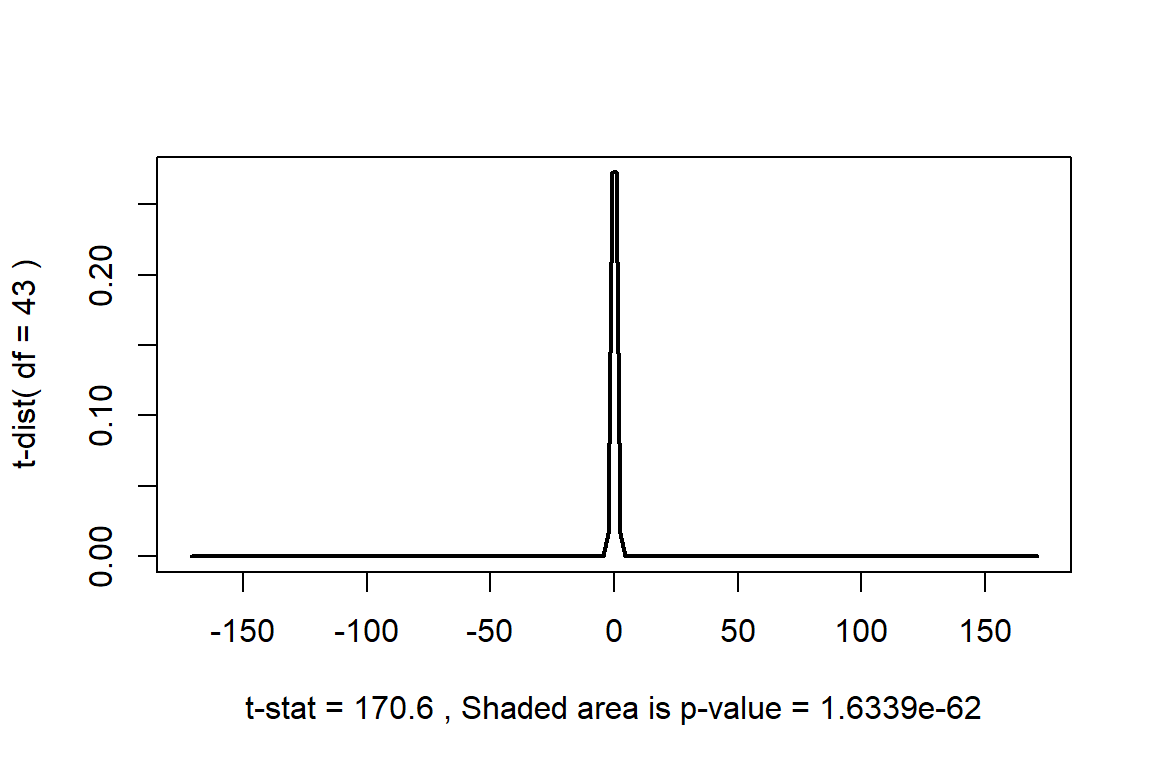Hypothesis test 1. ’’ •$H_0: $versus$H_A: $2. Let $$\alpha=0.05$$, the significance level of the test and the Type-I error probability if the null hypothesis is true. 3.$t_{s} = $. 4.$p = $, this is the observed significance of the test. 5. Because$p = $, … Also, given your conclusion, state whether you could have made a Type-I or Type-II error. # Earth’s water example ## Questions to answer 1. (3 p) In class we sampled the beach ball and observed 20 of 30 observations were water. Conduct a hypothesis test to determine whether the proportion of water on the beach ball is different from the amount of water on the earth’s surface (71%). ## notes for prop.test() and binom.test() # x = number of "successes" # n = total sample size #n = 2 #x = 1 x = 21 n = 21 + 14 dat.globe <- data.frame(type = c("Water", "Land"), freq = c(x, n - x), prop = c(x, n - x) / n) dat.globe ## type freq prop ## 1 Water 21 0.6 ## 2 Land 14 0.4 # binom.test() is an exact test for a binomial random variable b.summary <- binom.test(x = x, n = n, p = 0.5, conf.level = 0.95) b.summary ## ## Exact binomial test ## ## data: x and n ## number of successes = 21, number of trials = 35, p-value = 0.3105 ## alternative hypothesis: true probability of success is not equal to 0.5 ## 95 percent confidence interval: ## 0.4211177 0.7612919 ## sample estimates: ## probability of success ## 0.6 library(ggplot2) p <- ggplot(data = subset(dat.globe, type == "Water"), aes(x = type, y = prop)) p <- p + geom_hline(yintercept = c(0, 1), alpha = 1/4) p <- p + geom_bar(stat = "identity") p <- p + geom_errorbar(aes(min = b.summary$conf.int, max = b.summary$conf.int), width=0.25) p <- p + geom_hline(yintercept = 0.71, colour = "red") p <- p + scale_y_continuous(limits = c(0, 1)) p <- p + coord_flip() # flip the x and y axes for horizontal plot print(p)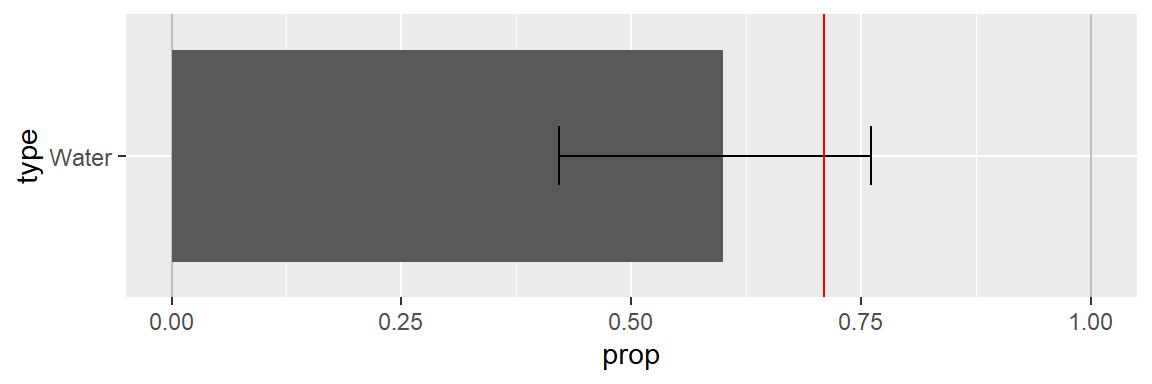Hypothesis test 1. ’’ •$H_0: $versus$H_A: $2. Let $$\alpha=0.05$$, the significance level of the test and the Type-I error probability if the null hypothesis is true. 3.$t_{s} = $. 4.$p = $, this is the observed significance of the test. 5. Because$p = $, … Also, given your conclusion, state whether you could have made a Type-I or Type-II error. # African countries in the UN example Previously in class we collected data using a randomized experiment. We provided a priming number (X = 10 or 65, not actually a random number) then asked you two questions: 1. Do you think the percentage of countries represented in the United Nations that are from Africa is higher or lower than X? 2. Give your best estimate of the percentage of countries represented in the United Nations that are from Africa. The data were compiled into a google doc which we read in below. # install.packages("gsheet") library(gsheet) dat.UN.Africa.url <- "docs.google.com/spreadsheets/d/1PkjIygZdtlopH6kvyle-KeoECsycHWyCaya92erlvaE" dat.UN.Africa <- gsheet2tbl(dat.UN.Africa.url) %>% as.data.frame() %>% na.omit() %>% mutate( PrimingNumber = factor(PrimingNumber) , HighLow = factor(HighLow) ) %>% filter( Class == "F19" ) str(dat.UN.Africa) ## 'data.frame': 69 obs. of 4 variables: ##$ PrimingNumber: Factor w/ 2 levels "10","65": 2 2 2 2 1 1 1 1 1 2 ...
##  $HighLow : Factor w/ 2 levels "H","L": 2 2 2 2 1 1 1 1 1 2 ... ##$ UN_Percentage: num  3 25 30 34 28 15 26 14 35 20 ...
##  $Class : chr "F19" "F19" "F19" "F19" ... Here are some summaries and plots. ## If we create a summary data.frame with a similar structure as our data, then we ## can annotate our plot with those summaries. # calculate the estimated mean and order M then F est.mean <- as.numeric(by(dat.UN.Africa$UN_Percentage, dat.UN.Africa$PrimingNumber, mean)) # combine true US mean with our estimated mean mean.UN.Africa <- data.frame(PrimingNumber = rev(unique(dat.UN.Africa$PrimingNumber))
, UN_Percentage = est.mean)
mean.UN.Africa <-
mean.UN.Africa %>%
mutate(
PrimingNumber = factor(PrimingNumber)
)
mean.UN.Africa
##   PrimingNumber UN_Percentage
## 1            10      17.97222
## 2            65      28.15152
# histogram using ggplot
p <- ggplot(dat.UN.Africa, aes(x = UN_Percentage))
p <- p + geom_histogram(binwidth = 4)
p <- p + geom_rug()
p <- p + geom_vline(data = mean.UN.Africa, aes(xintercept = UN_Percentage), colour = "red")
p <- p + facet_grid(PrimingNumber ~ .)
print(p)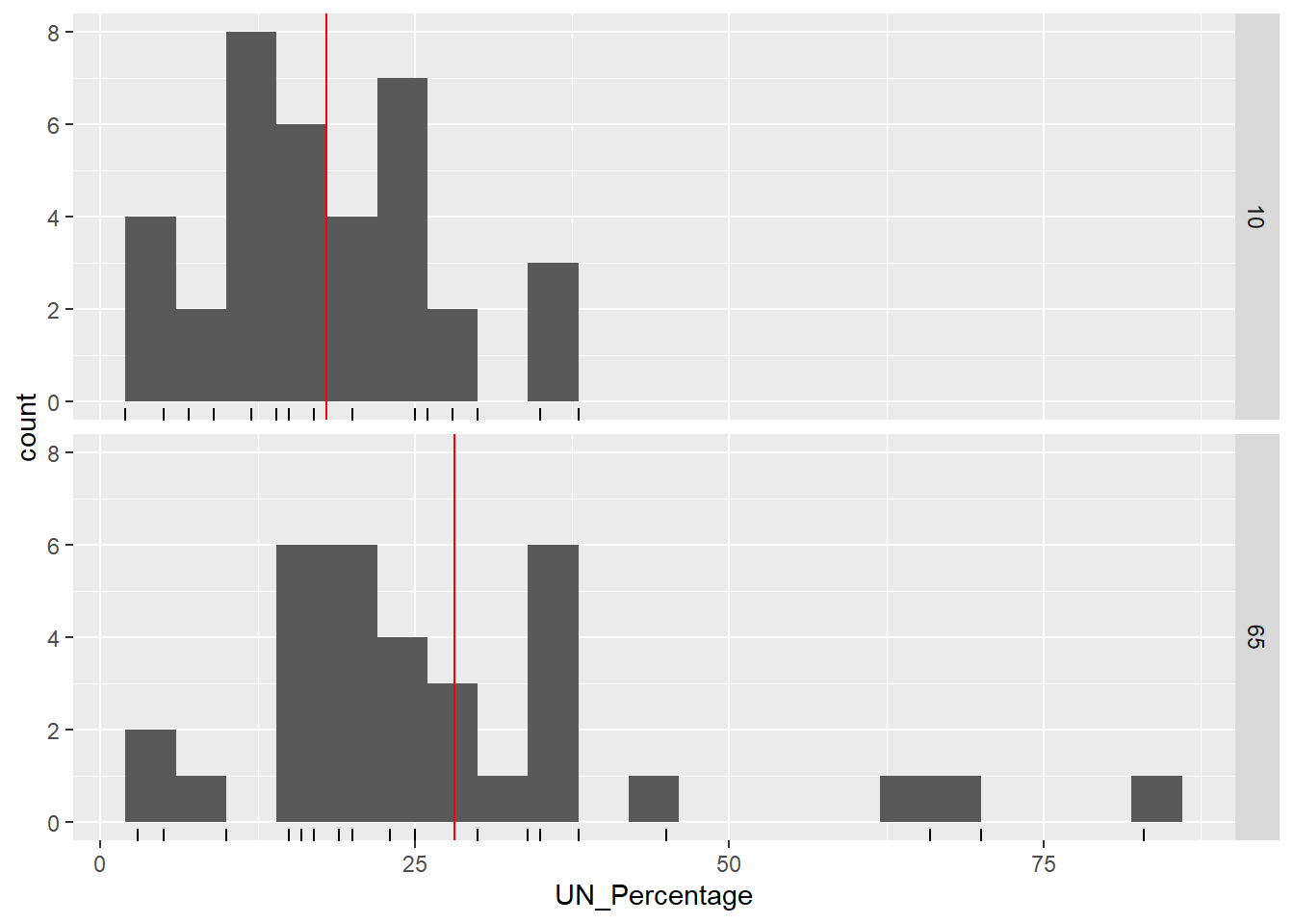p <- ggplot(dat.UN.Africa, aes(x = UN_Percentage, fill=PrimingNumber))
p <- p + geom_histogram(binwidth = 4, alpha = 0.5, position="identity")
p <- p + geom_rug()
p <- p + geom_vline(data = mean.UN.Africa, aes(xintercept = UN_Percentage, colour = PrimingNumber, linetype = PrimingNumber))
p <- p + geom_rug(aes(colour = PrimingNumber), alpha = 1/2)
print(p)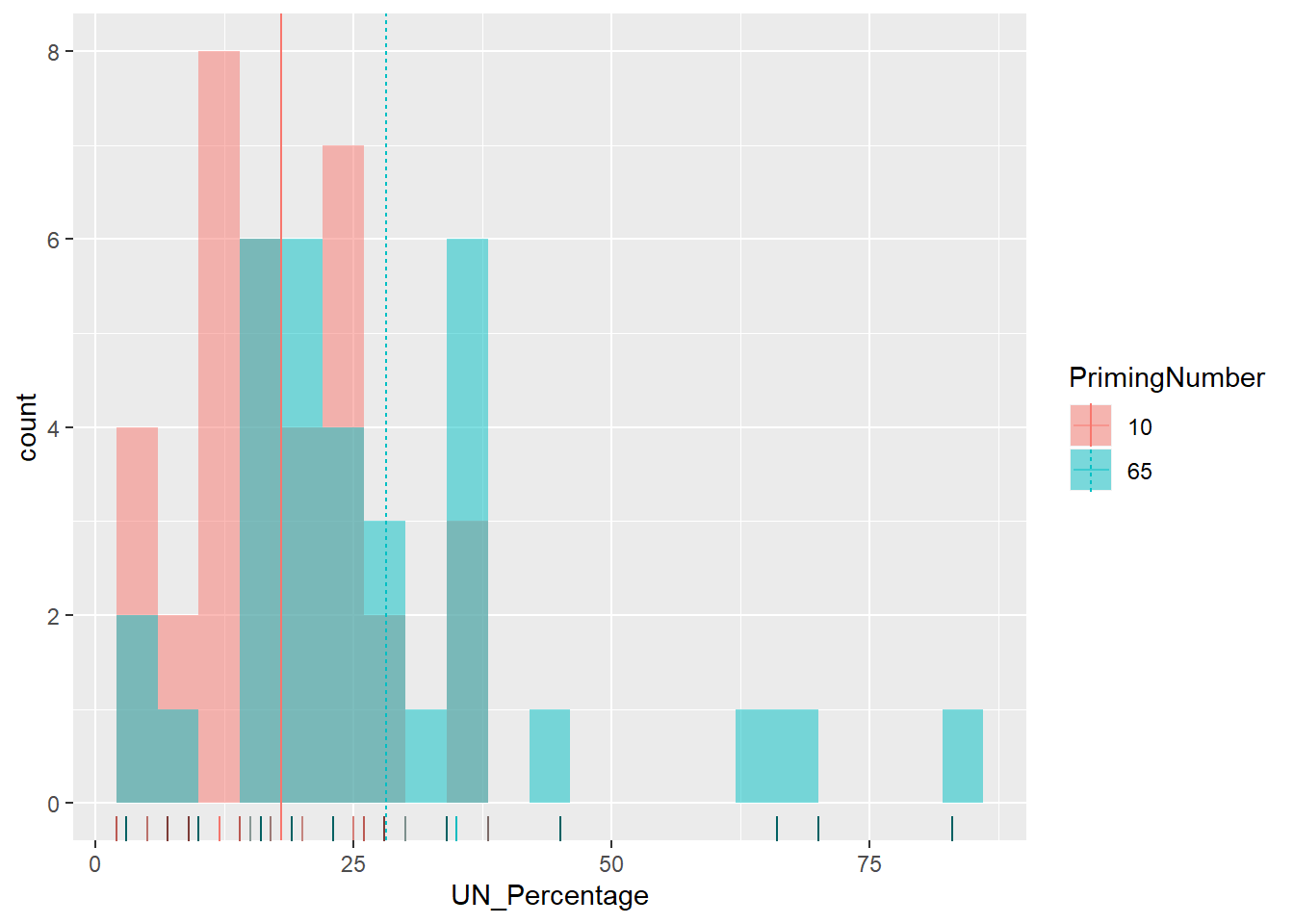A priori, before we observed the data, we hypothesized that those who were primed with a larger number (65) would provide a higher percentage (UN_Percentage) than those with the lower number (10). Therefore, this is a one-sided test.

1. (4 p) Set up the two-sample t-test and state the conclusions.
# two-sample t-test
t.summary.UN <- t.test(UN_Percentage ~ PrimingNumber, data = dat.UN.Africa
, alternative = "less")
t.summary.UN
##
##  Welch Two Sample t-test
##
## data:  UN_Percentage by PrimingNumber
## t = -2.9877, df = 47.438, p-value = 0.00222
## alternative hypothesis: true difference in means is less than 0
## 95 percent confidence interval:
##       -Inf -4.463638
## sample estimates:
## mean in group 10 mean in group 65
##         17.97222         28.15152
t.dist.pval(t.summary.UN)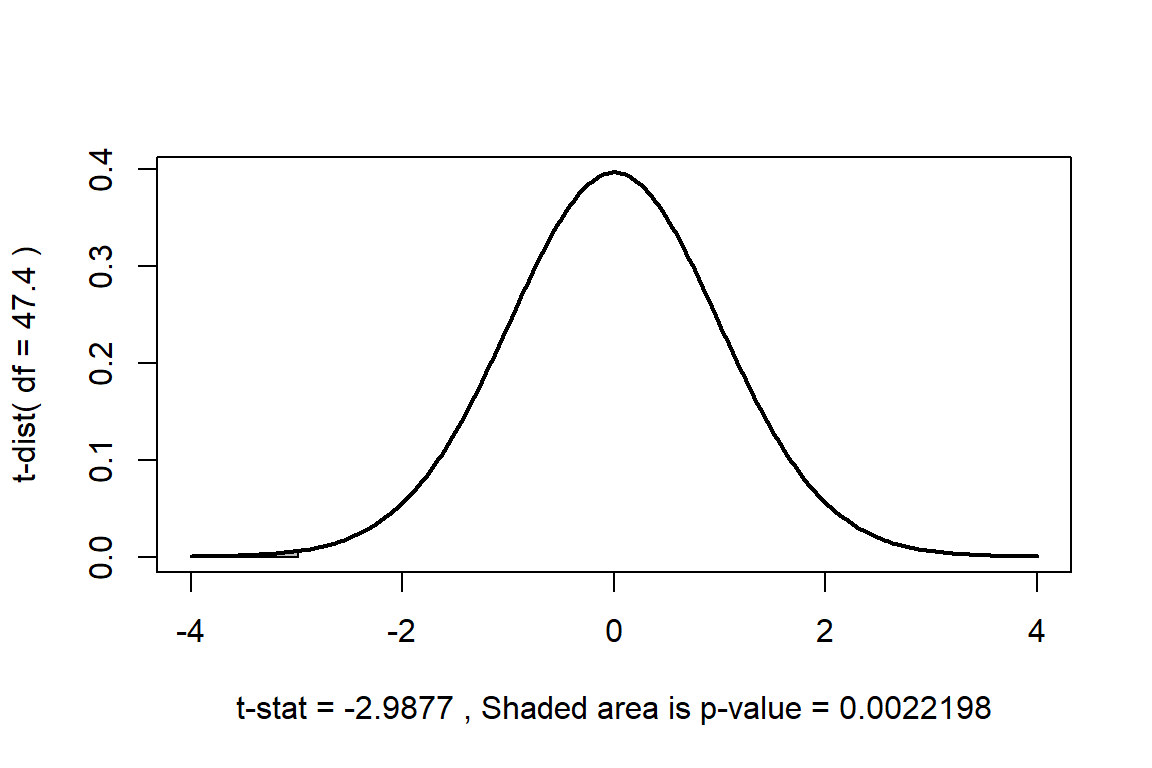Hypothesis test

1. ’’

• $H_0:$ versus $H_A:$
2. Let $$\alpha=0.05$$, the significance level of the test and the Type-I error probability if the null hypothesis is true.

3. $t_{s} =$.

4. $p =$, this is the observed significance of the test.

5. Because $p =$, …

Also, given your conclusion, state whether you could have made a Type-I or Type-II error.